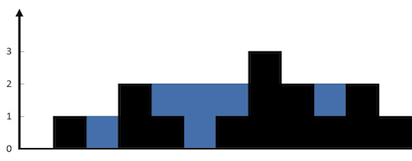## 题目``````输入: [0,1,0,2,1,0,1,3,2,1,2,1]

## 解法

### 动态编程

``````left-max - 每一列左边的最大值
right-max - 每一列右边的最大值``````
• 先从左到右遍历，得到每一列左边的最大值
• 再从右到左遍历，得到每一列右边的最大值
• 最后在从左到右遍历，将`当前列的高度``该列左边最大值``该列右边最大值`做比较，只有在`当前列的高度`同时小于`该列左边最大值``该列右边最大值`，当前列才能接水。接的水数量 = min(`该列左边最大值`, `该列右边最大值`) - `当前列的高度`
``````class Solution:
def trap(self, height: List[int]) -> int:
l = len(height)
left_max, right_max =  * l,  * l
# 先从左到右遍历，得到每一列左边的最大值
for i in range(1, l):
left_max[i] = max(height[i - 1], left_max[i - 1])
# 再从右到左遍历，得到每一列右边的最大值
for i in range(l - 2, -1, -1):
right_max[i] = max(height[i + 1], right_max[i + 1])
ants = 0
for i in range(l):
if height[i] < left_max[i] and height[i] < right_max[i]:
ants += min(left_max[i], right_max[i]) - height[i]
return ants``````

### 双指针

``````left_max - 左边的最大值
right_max - 右边的最大值
left - 从左往右处理的当前列
right - 从右往左处理的当前列``````

``````class Solution:
def trap(self, height: List[int]) -> int:
i, j = 0, len(height) - 1
left_max, right_max = 0, 0
ants = 0
while left <= right:
if left_max < right_max:
if height[left] < left_max:
ants += left_max - height[left]
else:
left_max = height[left]
left += 1
else:
if height[right] < right_max:
ants += right_max - height[right]
else:
right_max = height[right]
right -= 1
return ants``````
Last modification：May 19th, 2020 at 10:05 am